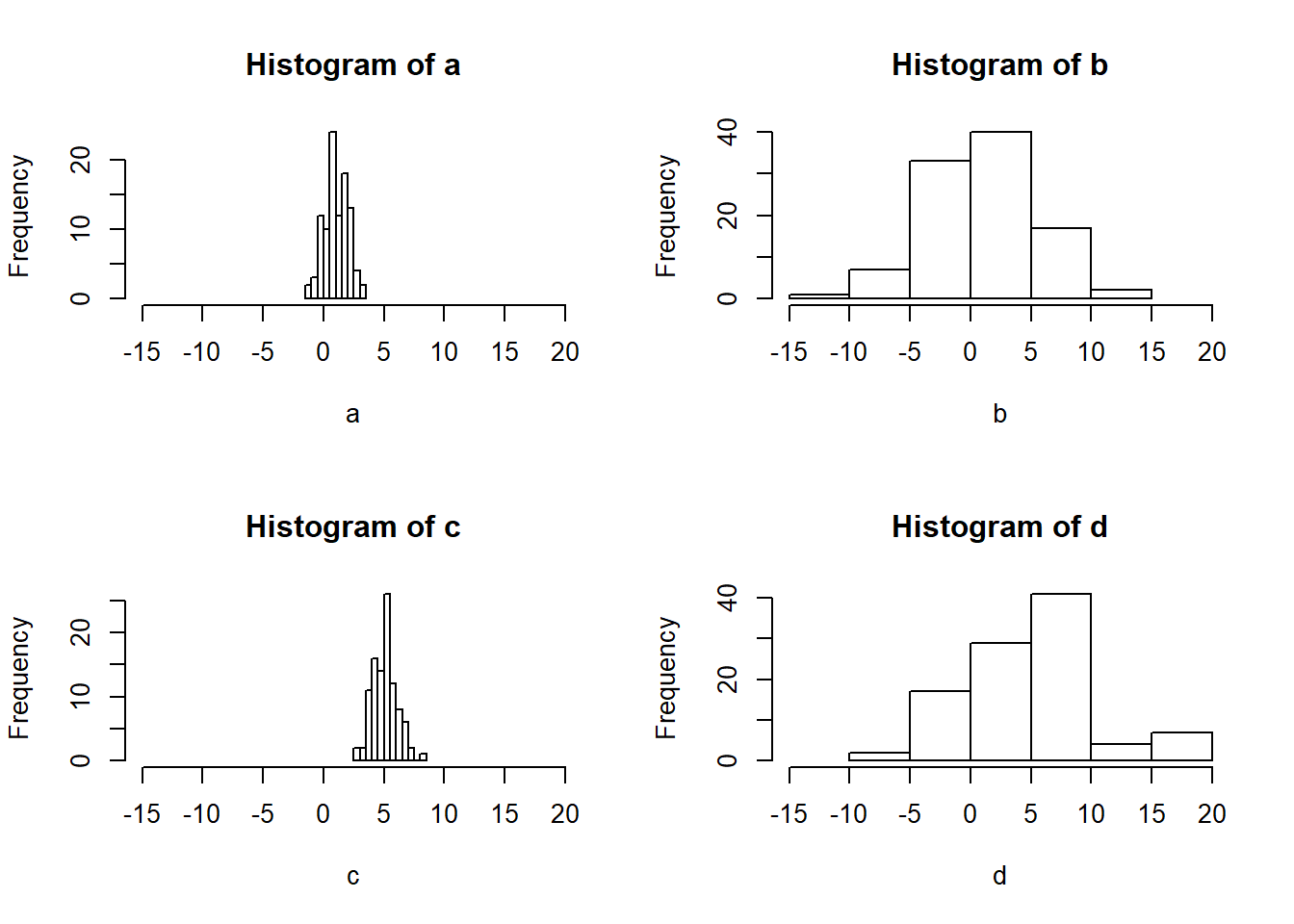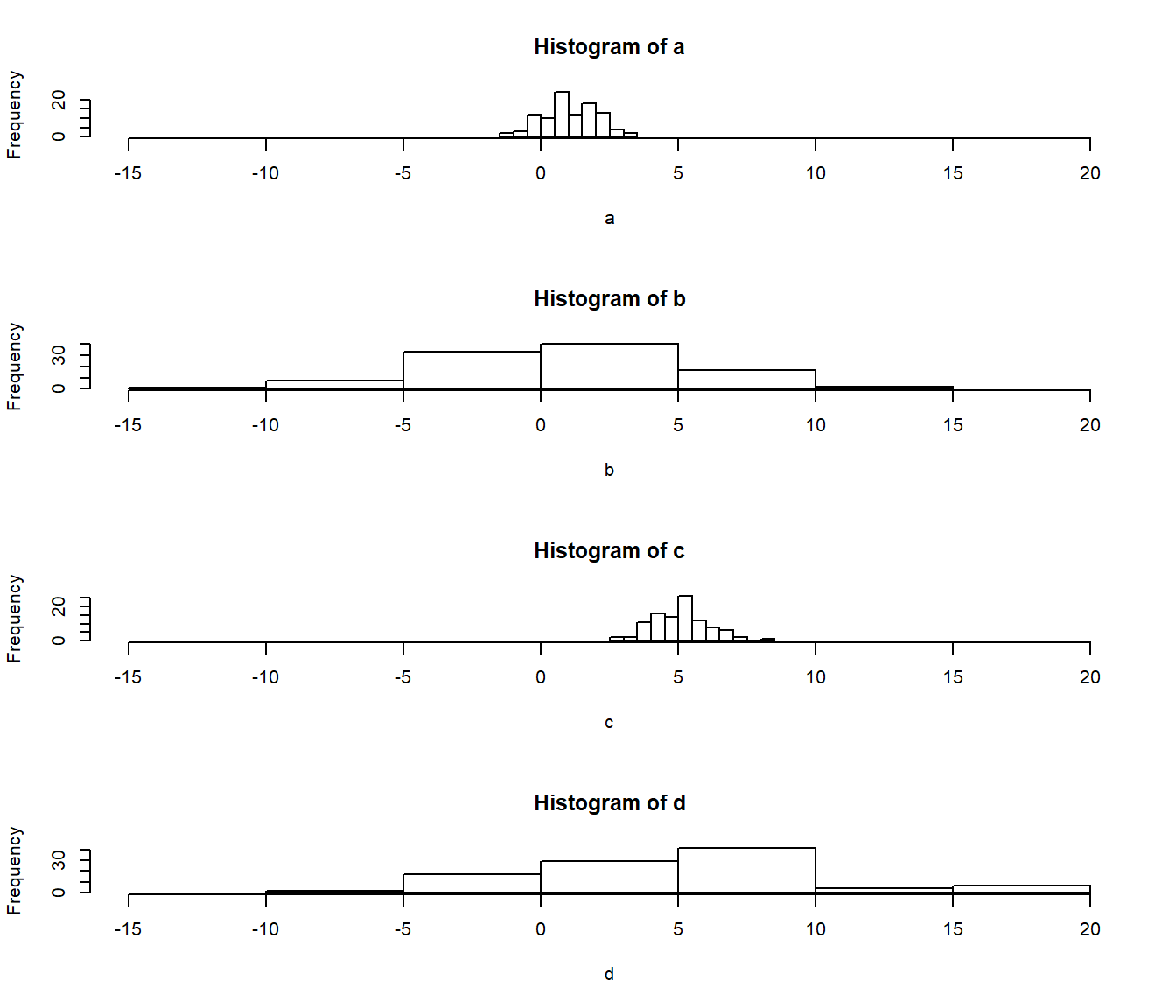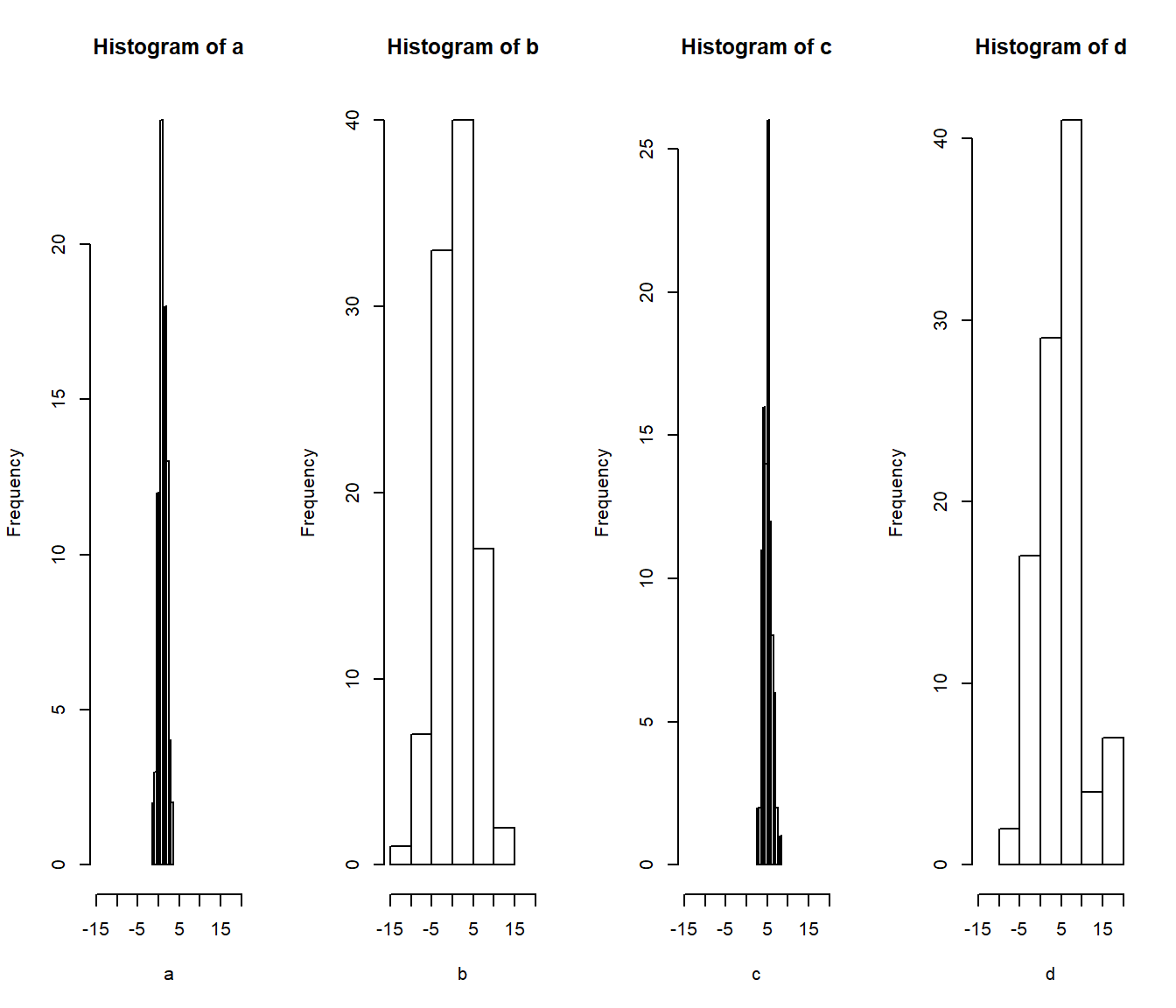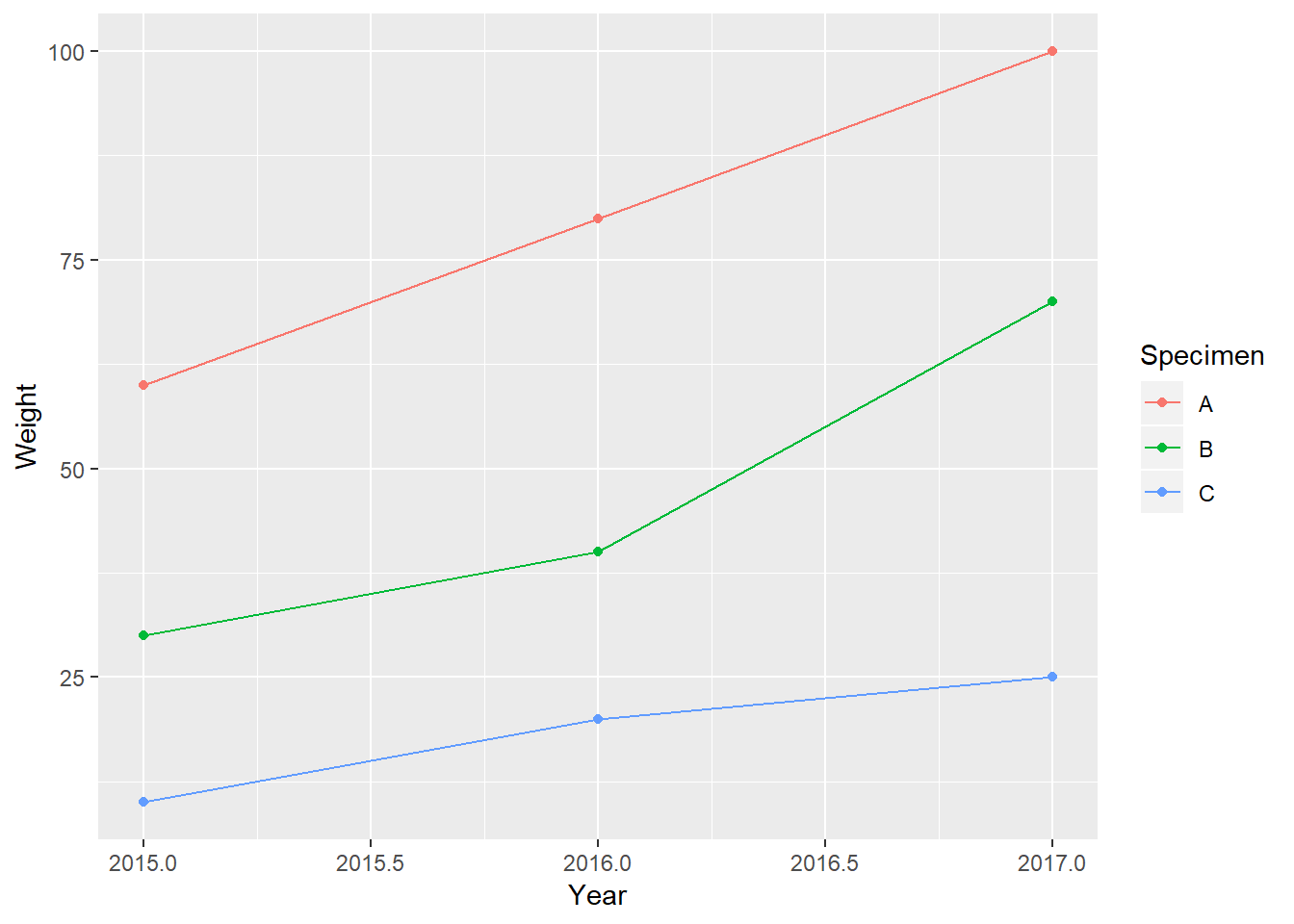## Upcoming Assignments/Quizzes

Assignments Open Time Due Time
Group Data Visualization Friday, Sept 21 (1:00 am) Sunday, Sept 30 (11:55 pm EST)
Module 5 Data Quiz Friday, Sept 28 (1:00 am EST) Sunday, Sept 30 (11:55 pm EST)
Module 5 Conceptual Quiz Friday, Sept 28 (1:00 am EST) Sunday, Sept 30 (11:55 pm EST)

## Notes from Discussion Board/Office Hours

### Multiple plots on one graph using par() and mfrow

Sometimes we may want to put multiple plots on the same graph (or graphing area). To do this, we will need to set the graphical parameters using the par(). There are many parameters we can set with using arguments inpar(), including setting the margins (with mar) and how to arrange multiple plots on the same graphic area with mfrow. The mfrow arugment takes a vector of length 2, which determines the number of rows and then the number of columns. In practice this works as par(mfrow = c(# of rows, # of columns)).

Here are some examples with histograms:

# Generate some data for histograms
a <- rnorm(100, 1, 1)
b <- rnorm(100, 1, 5)
c <- rnorm(100, 5, 1)
d <- rnorm(100, 5, 5)
# Make a 2x2 graph of the four histograms
par(mfrow = c(2,2))
hist(a, xlim = c(-15, 20))
hist(b, xlim = c(-15, 20))
hist(c, xlim = c(-15, 20))
hist(d, xlim = c(-15, 20))# Make a 4x1 graph (stacked on top) of the four histograms
par(mfrow = c(4,1))
hist(a, xlim = c(-15, 20))
hist(b, xlim = c(-15, 20))
hist(c, xlim = c(-15, 20))
hist(d, xlim = c(-15, 20))# Make a 1x4 graph (side-by-side) of the four histograms
par(mfrow = c(1,4))
hist(a, xlim = c(-15, 20))
hist(b, xlim = c(-15, 20))
hist(c, xlim = c(-15, 20))
hist(d, xlim = c(-15, 20))Note that whenever you set par(), R is going to use those graphic parameters for all of the plots you generate. If you want to change this behavior, you must change the parameters (for example: par(mfrow = c(1,1))).

### Reshaping data with tidyr::gather() and tidyr::spread()

A good rule of thumb for organizing data in a spreadsheet is that each row should represent an individual observation. Sometimes, though, you may see data like this:

Year A B C
2017 100 70 25
2016 80 40 20
2015 60 30 10

Where A, B, C are different specimens, and the value in each cell is the weight of that specimen in that particular year. Organizing data this way may make sense (especially depending on how it is collected), but it violates our rule and can make it diffult to analyze and/or visualize. Thankfully, we can use the gather() from the tidyr package to transform the data so that each row will be a single observation:

# You may need to install the package first
library(tidyr)
df_long <- gather(df, key = "Specimen", value = "Weight", A, B, C)
Year Specimen Weight
2017 A 100
2016 A 80
2015 A 60
2017 B 70
2016 B 40
2015 B 30
2017 C 25
2016 C 20
2015 C 10

This function will transform the data into the “long format”. To use this function, you specify the dataframe, then name for the “key” (column names) and the “value” (row values), then list the columns you want to gather. This makes it easier to plot the data using the ggplot2 package:

library(ggplot2)
## Warning: package 'ggplot2' was built under R version 3.5.1
ggplot(df_long, aes(x = Year, y = Weight, color = Specimen)) +
geom_point() +
geom_line()We can go back to the “wide format” using the spread() function:

df_wide <- spread(df_long, key = "Specimen", value = "Weight")
Year A B C
2015 60 30 10
2016 80 40 20
2017 100 70 25

### Other notes

• For the group assignment, one person will make one submission for the whole group

• We will take more about the T distribution more in future modules, the take-home for this lesson is to note the difference between using parameters versus estimates (of parameters), and what happens when we increase or decrease the number of samples in our estimate